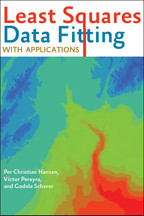Back to Results# Least Squares Data Fitting with Applications

Per Christian Hansen, Víctor Pereyra, and Godela Scherer

Publication Date
Request Exam CopyRequest Review Copy

A lucid explanation of the intricacies of both simple and complex least squares methods.

As one of the classical statistical regression techniques, and often the first to be taught to new students, least squares fitting can be a very effective tool in data analysis. Given measured data, we establish a relationship between independent and dependent variables so that we can use the data predictively. The main concern of Least Squares Data Fitting with Applications is how to do this on a computer with efficient and robust computational methods for linear and nonlinear relationships. The...

A lucid explanation of the intricacies of both simple and complex least squares methods.

As one of the classical statistical regression techniques, and often the first to be taught to new students, least squares fitting can be a very effective tool in data analysis. Given measured data, we establish a relationship between independent and dependent variables so that we can use the data predictively. The main concern of Least Squares Data Fitting with Applications is how to do this on a computer with efficient and robust computational methods for linear and nonlinear relationships. The presentation also establishes a link between the statistical setting and the computational issues.

In a number of applications, the accuracy and efficiency of the least squares fit is central, and Per Christian Hansen, Víctor Pereyra, and Godela Scherer survey modern computational methods and illustrate them in fields ranging from engineering and environmental sciences to geophysics. Anyone working with problems of linear and nonlinear least squares fitting will find this book invaluable as a hands-on guide, with accessible text and carefully explained problems.

Included are
• an overview of computational methods together with their properties and advantages
• topics from statistical regression analysis that help readers to understand and evaluate the computed solutions
• many examples that illustrate the techniques and algorithms

Least Squares Data Fitting with Applications can be used as a textbook for advanced undergraduate or graduate courses and professionals in the sciences and in engineering.

Reviews

## Reviews

Least Square Data fitting with Applications is a book that will be of great practical use to anyone whose work involves the analysis of data and its modeling. It offers a great deal of information that can be a s valuable in the lecture theater as in the lab or office.

Least squares remains a key topic in scientific computing, serving as a vital bridge between data and models. This book describes many interesting aspects of this problem class, including its statistical foundations, algorithms for solving both linear and nonlinear models, and its applications to many disciplines. The authors convey both the rich history of the subject and its ongoing importance.

## Book Details

Publication Date
Status
Available
Trim Size
6
x
9
Pages
328
ISBN
9781421407869
Illustration Description
9 halftones, 8 line drawings, 46 graphs
Subject

Preface
Symbols and Acronyms
Chapter 1. The Linear Data Fitting Problem
1.1. Parameter estimation, data approximation
1.2. Formulation of the data fitting problem
1.3. Maximum likelihood estimation
1.4. The

Preface
Symbols and Acronyms
Chapter 1. The Linear Data Fitting Problem
1.1. Parameter estimation, data approximation
1.2. Formulation of the data fitting problem
1.3. Maximum likelihood estimation
1.4. The residuals and their properties
1.5. Robust regression
Chapter 2. The Linear Least Squares Problem
2.1. Linear least squares problem formulation
2.2. The QR factorization and its role
2.3. Permuted QR factorization
Chapter 3. Analysis of Least Squares Problems
3.1. The pseudoinverse
3.2. The singular value decomposition
3.3. Generalized singular value decomposition
3.4. Condition number and column scaling
3.5. Perturbation analysis
Chapter 4. Direct Methods for Full-Rank Problems
4.1. Normal equations
4.2. LU factorization
4.3. QR factorization
4.4. Modifying least squares problems
4.5. Iterative refinement
4.6. Stability and condition number estimation
4.7. Comparison of the methods
Chapter 5. Direct Methods for Rank-Deficient Problems
5.1. Numerical rank
5.2. Peters-Wilkinson LU factorization
5.3. QR factorization with column permutations
5.4. UTV and VSV decompositions
5.5. Bidiagonalization
5.6. SVD computations
Chapter 6. Methods for Large-Scale Problems
6.1. Iterative versus direct methods
6.2. Classical stationary methods
6.3. Non-stationary methods, Krylov methods
6.4. Practicalities: preconditioning and stopping criteria
6.5. Block methods
Chapter 7. Additional Topics in Least Squares
7.1. Constrained linear least squares problems
7.2. Missing data problems
7.3. Total least squares (TLS)
7.4. Convex optimization
7.5. Compressed sensing
Chapter 8. Nonlinear Least Squares Problems
8.1. Introduction
8.2. Unconstrained problems
8.3. Optimality conditions for constrained problems
8.4. Separable nonlinear least squares problems
8.5. Multiobjective optimization
Chapter 9. Algorithms for Solving Nonlinear LSQ Problems
9.1. Newton's method
9.2. The Gauss-Newton method
9.3. The Levenberg-Marquardt method
9.5. Iteratively reweighted LSQ algorithms for robust data fitting problems
9.6. Variable projection algorithm
9.7. Block methods for large-scale problems
Chapter 10. Ill-Conditioned Problems
10.1. Characterization
10.2. Regularization methods
10.3. Parameter selection techniques
10.4. Extensions of Tikhonov regularization
10.5. Ill-conditioned NLLSQ problems
Chapter 11. Linear Least Squares Applications
11.1. Splines in approximation
11.2. Global temperatures data fitting
11.3. Geological surface modeling
Chapter 12. Nonlinear Least Squares Applications
12.1. Neural networks training
12.2. Response surfaces, surrogates or proxies
12.3. Optimal design of a supersonic aircraft
12.4. NMR spectroscopy
12.5. Piezoelectric crystal identification
12.6. Travel time inversion of seismic data
Appendix A: Sensitivity Analysis
A.1. Floating-point arithmetic
A.2. Stability, conditioning and accuracy
Appendix B: Linear Algebra Background
B.1. Norms
B.2. Condition number
B.3. Orthogonality
C.1. Convergence rates
C.2. Multivariable calculus
Appendix D: Statistics
D.1. Definitions
D.2. Hypothesis testing
References
Index

Author Bios
Featured Contributor

## Per Christian Hansen

Featured Contributor

## Víctor Pereyra

Víctor Pereyra is a consulting professor of energy resources engineering at Stanford University and was a principal at Weidlinger Associates, Los Altos, California.
Featured Contributor